# Optimal Learning Rate in Gradient Descent and Second Derivatives

## 1: What is a Gradient of a function, geometrically?

Why do we need to know that?

Brief summary of gradient descent: If you are on a hillside and you want to get to the bottom of the valley as quickly as possible, you don’t follow the contour, that would keep your height the same. The iterative procedure is to go to the next lower contour, taking the shortest and steepest step possible, or equivalently, go to the lowest possible contour keeping your step size the same. Now you can imagine the contours as (locally) parallel sets of curves, see the figure below. But a step is a direction and length. Intuitively, we want to step “perpendicular” to the contour: to the closest point on the lower contour. How do we show this and how do we get “mathematically” from a set of almost parallel curves representing the function to a direction in which to reduce the function?

Some places to read about Gradient Descent, in ascending order: https://medium.com/deep-math-machine-learning-ai/chapter-1-2-gradient-descent-with-math-d4f2871af402, …

In many of the introductory lectures on Machine Learning, for extremizing a function of one variable, it is suggested that the learning rate be set at the inverse of the second derivative. Generalizing this to multiple dimensions involves “…Hessian…”.

If you are comfortable with differential calculus, you can skip directly to the end. The general argument goes as follows:

1. In any valley of the function f you are trying to minimize, expand the function as a (multi-dimensional) Taylor series about some arbitrary initial point x0.
2. Approximate the function by keeping only terms up to quadratic in the displacement (x x0).
3. Extremize this approximation by setting it’s derivative to 0.
4. Solve for the displacement that takes you from x to the minimum of the quadratic approximation to f in one step.
5. This yields that the ideal step is the inverse of the Hessian times (the negative of) the gradient.

In order to get to the business about second derivatives and Hessians, it helps (me) to understand the following.

A (real) function is a mapping from a space into the Reals. If it is a function on 2 dimensions, then given any point (x,y) in two dimensions the function gives you back a real number. This can be done in many ways:

• as an array of values (x, y, f(x,y)),
• a numerical means of calculation or
• in so-called “closed” functional form e.g. f(x, y) = y*sin(3*x) (as a shorthand for the numerical computation).

What does a function look like? You can show it as a surface in 3 dimensions where z = f(x,y), or as a topographic map in 2D: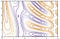Representation of a function in 2D

where I’ve left off the contour values.

What is its gradient? The gradient of the function is the contour plot! In this case it is not a constant, just as a fluid flow may have vectors pointing in different directions at different points. But the gradient is not a vector, it is a co-vector.

Great, so what is a covector? Given a function, its gradient can be constructed naturally, we just did it above! A co-vector is a sum of gradients (but it may not itself be a gradient).

We know what a vector v^a looks like: it is an arrow with direction and magnitude. We even know how to add vectors: lay them head to tail and join the first tail to the last head:Vector addition

A vector field is an arrow at every point, if you have flow lines, then it is the tangent vectors at every point on the lines: https://en.wikipedia.org/wiki/Vector_field

We’ve already visualized the gradient field, the contour plot above. What does a co-vector w_a (at a point) look like? It looks like two parallel planes: at a given point the tangent plane at that point and the one on the next contour. In 2D, it looks like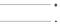A co-vector

Why is it called a co-vector and what is it? A co-vector is a linear map from vectors to (in this case) Reals. This map is written as w_a v^a = w(v) = <w, v> in R. How do we calculate it? It is defined as the number of w’s needed to span the vector: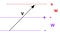Action of a co-vector on a vector

In this case w(v) = 2, since you have to place two w’s “head-to-toe” to span v. Two points here: first, two w’s “head-to-toe” is NOT 2*w, and second, w(v) depends on the magnitudes of v and w, as well as their relative direction.

Covector addition: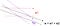Covector addition

As the covector becomes larger, the lines (or planes) get closer! Note that this also makes sense intuitively in a contour map: the steepest parts are where the contours are closely spaced.

The main points so far are the following, for minimizing a function using gradient descent:

• We want to know in which direction and by how much to step. This is a displacement and hence a vector, with an “upper” index, or a tensor of type (1,0), or a column matrix
• We know the contours of the function and hence its gradient
• But the gradient is a simply (locally) the location of the next contour line of the function and hence a co-vector, which has a “lower” index, or a tensor of type (0,1) or a row matrix
• How do we get from a row matrix to a column matrix?
• We need a row of columns, or a tensor of type (2,0), i.e., something like T^{ab}, so that v^a = T^{ab} w_b.
• Geometrically, we need to convert contour lines into arrows.
• We think this is obvious, because we move in (to a very good approximation) Euclidean space.
• But in the space of weights x, where the loss is a function of the weights which we are trying to minimize, there is no natural metric, even after normalization.

In matrix algebra, the identity matrix is a tensor of type (1,1), a row of columns or a column of rows. The “transpose” operation that allows us to go from a column vector to a row co-vector implicitly assumes and uses a Euclidean type (2,0) tensor ((1,0,0), (0,1,0), (0,0,1)), which is a “row of rows”. This is NOT the identity matrix, which is a tensor of type (1,1).

Let’s figure out the ideal step, — or equivalently, the learning rate — in 1-dimension first.

## 2: Ideal Learning Rate for a 1 Dimensional Quadratic

Consider a quadratic as an approximation to the loss function to be minimized (in one particular valley), in a one dimensional space {x}, and assume we already know its functional form.

The Loss or function to be minimized is (note that we are now using y for the target function and not a variable)

y = f(x) = a* x**2 + b*x + c

its gradient or derivative at any point x is

dy(x) = f’(x) = 2*a*x + b

and its second derivative is

ddy = f’’(x) = 2*a

The minimum of this function is at

X = arg(min(y)) = argmin(y)

found by setting the first derivative of y, dy(X) = 0.

So we get X = -b/(2*a)

Now imagine that we are starting at some arbitrary initial point x0 and we want to get to X. Gradient descent says:

• evaluate the derivative at x0: dy(x0)
• step in the opposite direction to x1 = x0 + δx, where δx = -α * dy(x0)
• But by how much? This is the learning rate α

How do we optimize α so we reach bottom in the minimum number of steps? You can say, we are starting at x0 and we want to get to X, we can do it in one step if we choose x1 = X, i.e.

δx = x1 — x0 = Xx0 = -(x0 + b/(2*a)).

The problem is, when we start at some arbitrary point x0, and we only have a way of calculating the value of the Loss function at any point, we have no idea what are a, b, c, not even X = -b/(2*a). This is akin to walking around on a hillside, in a dense fog, with only an altimeter, and facing the problem of reaching the valley bottom.

What we do know is how to compute y(x) for any x. So we can compute y(x0). We can also compute its derivative(s). How? We can compute y(x0 + ε) and by choosing ε “small enough”:

dy(x0) ~= (y(x0 + ε) — y(x0))/ ε

Is this enough for us to figure out how to get to the bottom? No, because if we only know the first derivative, we are approximating the loss as a straight line and the tangent line has no information about the location of the minimum: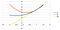Two quadratics tangent at a point.

Both the blue and the red curves have the same derivative at x = 1, but they have minima at -0.5 and + 0.25 respectively. Clearly, to get the minimum, we need to know how the curve curves.

So back to the quadratic approximation to the loss function. We know the step is related to the gradient via:

δx = — α * dy(x0)

we also know the gradient:

dy(x0) = f’(x) = 2*a*x0 + b

and we already figured out the ideal step

δx = x1 — x0 = Xx0 = -(x0 + b/(2*a))

Putting these together, we find that the learning rate

α = — δx/dy(x0) = 1/(2*a) = 1/ddy(x0) = inverse of the second derivative.

So the ideal step is given by

δx = — dy(x0) / ddy(x0)

Let’s generalize and derive this heuristic a bit more formally.

## 3: Approximate Ideal Step in 1 dimension

In the previous section we showed that if we want to find the minimum of a quadratic in one dimension using “Machine Learning”, specifically using gradient descent, the ideal learning rate is the inverse of the second derivative at the current point. In this post let’s try and generalize this to finding the local minimum of almost any curve y = y(x) in 1 dimension.

Expand y(x) in a Taylor series around the initial point x0:

y(x) ~= y(x0) + (xx0) * dy(x0) + (xx0)**2 * ddy(x0) / 2 + …

X = argmin(y(x)) is given by the first derivative = 0:

dy(x0) + (Xx0)*ddy(x0) ~= 0

=> δx = — dy(x0) / ddy(x0).

The step is in the opposite direction to the gradient (the first derivative), and the learning rate — or the factor by which the gradient is multiplied to find the ideal step- is the inverse of the second derivative.

This result is independent of the coordinate, but non-linear coordinate transformations will lead to different steps since the quadratic approximation to the “valley” in y(x) will be different in different coordinates.

Finally, let’s

## 4: Generalize to Multiple Dimensions

In the previous section we showed that the inverse of the second derivative is an approximation to the ideal learning rate for finding the minimum of almost any function in 1 dimension using Gradient Descent. In this post we generalize this result to multiple dimensions, and show how to get from a gradient co-vector to a vector step via the mysterious and missing tensor of type (0,2).

We have multiple weights we are trying to use to minimize some Loss function. So the weight space {x} is multiple dimensional and a point is given by the vector x or x^a. The Loss function y(x) can be expanded in Taylor series:

y(x) ~= y(x0) + δx dy(x0) + δx δx ddy(x0) / 2 + …

where dy = (∂y/∂x^i) d x^i, the gradient, is a co-vector, and δx is the increment change in the weights. In terms of components or indices:

y(x) ~= y(x0) + δx^i d_i y(x0) + δx^i δx^j H_{ij}(x0) / 2 + …

where H_{ij} = (∂/∂x^i) (∂/∂x^j) y

is the Hessian or the matrix of mixed partial derivatives of y.

To find the extremum, we set = 0 the gradient of y(x) w.r.t. x, and keep in mind that δx = xx0:

0 = d_i y(x) = d_i y(x0) + δx^j H_{ij}(x0)

which can be solved for the increment at the point x0:

δx^i = — H^{ij}(x0) d_j y(x0)

where H^{ij} with the upper indices is (a (0,2) tensor) the inverse of the Hessian:

H^{ik} H_{kj} = I^i_j

In words, the ideal increment is the inverse of the Hessian times (the negative of) the gradient.

The “learning rate” is actually a tensor.

Of course it is computationally a pain to calculate the O(D²) Hessian and the O() inverse at every step, but one approach might be to do it every few epochs and assume that the same quadratic approximation holds good for that duration.

Are there situations in which this can be simplified? Is there some sense in which the variables can be deemed to be “orthogonal” to each other and “normalized”? Yes, but the only measure of this is from the Hessian itself! If we know the Hessian is at least approximately diagonal and that its eigenvalues are approximately equal, then we could calculate the D-th root of its determinant and we have the inverse of the Hessian

H^{ij} = (detH)^{-D} diag(1,1,1 …)

This is the justification for using the inverse D-th root of the determinant of the Hessian as the scalar learning rate.

However, calculating the determinant is still almost O(), so we haven’t gained any computational simplicity.

How does this work for simple examples? (And I’ll bet all of you want to see some code.)

## More from Ranjeet Tate

I stop to miau to cats.